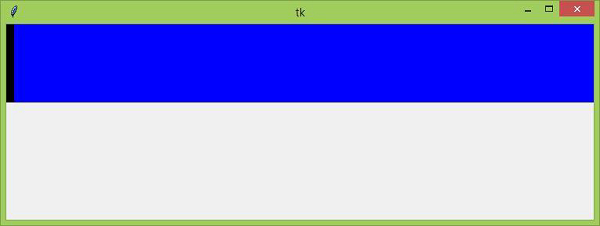# What does 'weight' do in tkinter?

In order to structure the layout of the widgets in an application, we generally use the tkinter grid system. A grid system comprises rows and columns in which widgets are aligned. If we want to configure any widgets, then we can use the grid row and columns properties.

Consider, if a widget is aligned such that there is extra space left, then we can add the weight property which enables the column to grow. A non-zero weight enables the column width to grow in the screen size if there is any extra space left. However, if the weight is zero, then the column width cannot grow. We can understand the weight property with an example by assigning some value to the weight property.

## Example

#Import tkinter library
from tkinter import *
#Create an instance of tkinter frame
win= Tk()
#Set the Geometry
win.geometry("750x250")
#Create two frames
frame1=Frame(win,background="black", width=10, height=100)
frame2=Frame(win,background="blue", width=10, height=100)
frame1.grid(row=0, column=0, sticky="nsew")
frame2.grid(row=0, column=1, sticky="nsew")
win.grid_columnconfigure(0, weight=0)
win.grid_columnconfigure(1, weight=1)
win.mainloop()

## Output

Executing the above code will display a window containing two frames with different colors. weight=0 disables the column width to grow with respect to its frame size. However, weight=1enables the column width to grow if there is any space in the frame.